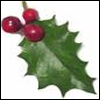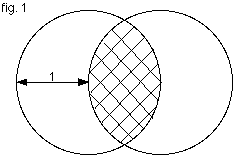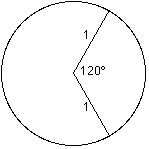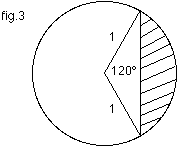#### You may also like### Giant Holly Leaf

Find the perimeter and area of a holly leaf that will not lie flat (it has negative curvature with 'circles' having circumference greater than 2πr).Given a square ABCD of sides 10 cm, and using the corners as centres, construct four quadrants with radius 10 cm each inside the square. The four arcs intersect at P, Q, R and S. Find the area enclosed by PQRS.### Get Cross

A white cross is placed symmetrically in a red disc with the central square of side length sqrt 2 and the arms of the cross of length 1 unit. What is the area of the disc still showing?

# Two Circles

##### Age 14 to 16 Challenge Level:

The required area is shown below in fig. $1$.To find the area it may help to consider just one of the circles and the sector created.

The angle in the sector is $120$ degrees. (See fig. $2$.)Hence the area of the sector is $\frac{120}{360} \times\pi \times1 \times1 = \frac{\pi}{3}$.

Now by considering the area of the triangle in fig. $3$, we can find the area of the shaded segment.The area of the triangle $= 0.5 \times\sin 120^{\circ} = \frac{\sqrt{3}}{4}$ So the area of the shaded segment $= \left(\frac{\pi}{3} - \frac{\sqrt{3}}{4}\right)$ square units. The area of the overlap is twice this amount which is : $2\left(\frac{\pi}{3} - \frac{\sqrt{3}}{4}\right) = \frac{2\pi}{3} - \frac{\sqrt{3}}{2}$ square units.